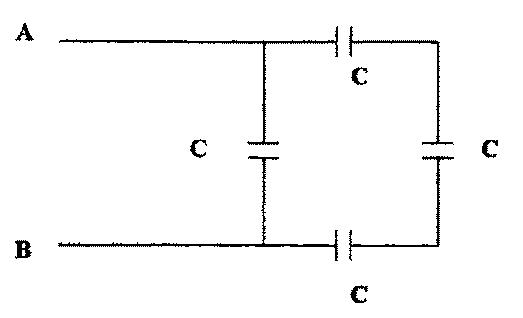## University of California, San Diego Physics 1b - Thermal Physics & Electromagnetism

 H. E. Smith Spring 2000Physics 1B - Tutorial #61. In the network above, each capacitor has a capacitance of 2µF.
• Calculate the equivalect capacitance of the network.
• If 10V is applied across A and B, what is the charge on each capacitor?

2. Your TA will hand you a "disk" capaccitor, which consists of a pair of circular metal disks, separated by mica insulation, then enclosed in a hard insulating shell. Estimate the capacitance of this capacitor if the "dielectric constant" of mica, k = 7.5 (= k.0).3. A capacitor made of a close pair of conducting plates, as shown above, is charged then discharged to produce a spark. The plates are charged a second time, and this time the plates are pulled further apart before being discharged. Will the second charge be (a) bigger than, (b) the same as, or (c) smaller than the first spark. Explain your reasoning.

4. A mountain lake is to be used as the water supply for an alpine village which lies a vertical distance h below the lake. The lake and village are to be connected by a system of pipes. How will the flow of water depend upon:
• the height, h, of the lake above the village.
• the diameter of the pipes.
• the length of the pipes (assuming that there is some form of viscous drag - friction).
What would happen if you put in two parallel pipes of the same length & diameter? What would happen if you doubled the length of the pipe? What does this have to do with electricity?5. Above is a diagram of the electrical system of an automobile. Which swithces (A, B, C, D, E, F) must be closed in order to
• a. Blow the horn.
• b. Turn on the headlights.
• c. Turn on the taillights.
• d. Turn on the parking lights.
• e. Turn on the dome light.
• f. When do the side marker lights go on?
Note that the whole body of the car, including the engine is wired together as a common ground. (Hecht: Ch. 19 #23, p. 749)Physics 1B HomeTutorials

Gene Smith